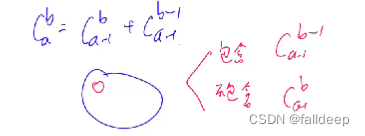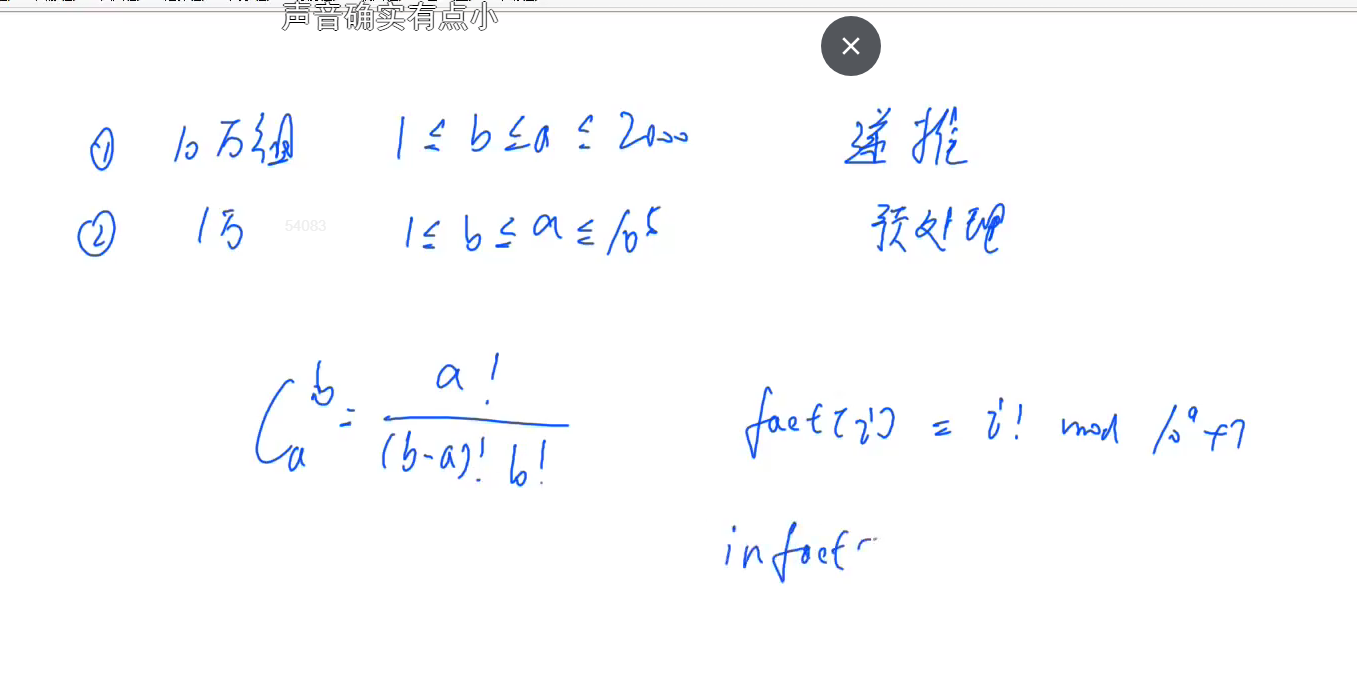# 类型1

1≤n≤10000,
1≤b≤a≤2000

``````3
3 1
5 3
2 2
``````

``````3
10
1``````

# 此时的参数为

1≤n≤10000,
1≤b≤a≤2000

c（a b）可以看作是从a个苹果中选取b个苹果，# 类型1代码

``````#include<iostream>

using namespace std;

int mod = 1e9 + 7;
const int N = 2010;
int f[N][N];
int n;

void init()
{
for(int i = 0; i < N; i ++)
for(int j = 0; j <= i; j ++)
if(!j) f[i][j] = 1;
else f[i][j] = (f[i - 1][j - 1] + f[i - 1][j]) % mod;

}

int main()
{
init();

cin >> n;
for(int i = 0; i < n; i ++)
{
int a, b;
cin >> a >> b;
cout << f[a][b] << endl;
}

return 0;
} ``````

# 类型2

1≤n≤100001≤n≤10000,
1≤b≤a≤105

# 类型2思路

a,b数据也较大，先预处理出各个数的阶乘，然后再模拟手工计算，利用公式算出结果。# 类型2代码

``````#include<iostream>

using namespace std;

typedef long long LL;
int p = 1e9 + 7;
const int N = 1e5 + 10;
int fact[N], infact[N];//阶乘和逆

int qmi(int a, int k, int p)
{
int res = 1;
while(k)
{
if(k & 1) res = (LL) res * a % p;
a = (LL) a * a % p;
k >>= 1;
}

return res;
}

int main()
{
int n;
cin >> n;

fact = infact = 1;
for(int i = 1; i < N; i ++)
{
fact[i] = (LL) fact[i - 1] * i % p;
infact[i] = (LL) infact[i - 1] * qmi(i, p - 2, p) % p;
}

while(n --)
{
int a, b;
cin >> a >> b;
int res;
res = (LL) fact[a] * infact[b] % p * infact[a - b] % p;
cout << res << endl;
}

return 0;
}``````